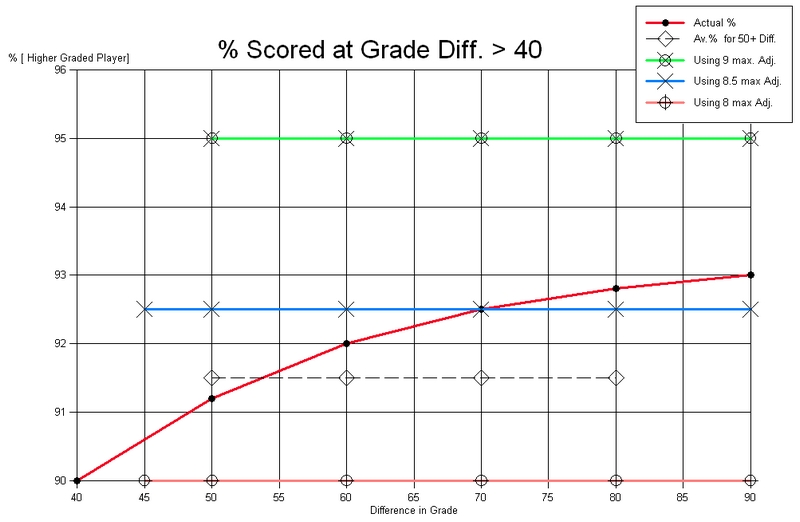Roger Tuddenham's proposed change to the Rating system.
Roger is proposing that we improve accuracy by using ratings to the nearest half [i.e. grades rounded to the nearest five, not ten as before]. See below.
Present system
(G.) RULES APPLICABLE TO HANDICAP GAMES
1.A player's grade is found by dividing his grade by 10, and rounding to the nearest whole one. e.g. 132 rating is 13, 85 rating is 9 2.
For each individual game an adjustment is given to the lower rated player as follows.
Difference in Rating             Lower Rated gets adjustment of...
1                                       2
2                                       4
3                                       6
4                                       8
5 or more                                9 (this is the maximum adjustment possible)

Proposed by Roger Tuddenham
1. A player's grade is found by dividing his grade by 10, and rounding to the nearest half. e.g. 132 rating is 13, 84 rating is 8.5, 113 is 11.5, 97 is 9.5
Difference in Rating              Lower Rated gets adjustment of...
0.5                                      1
1                                        2
1.5                                      3
2                                        4
2.5                                      5
3                                        6
3.5                                      7
4                                        8
4.5 or more                        8.5 (this is the maximum adjustment possible)

Note that If all six games in a match were 8.5 adjustment [or 8 adj], the points start would be 6 x 8.5 = 51, which gives 5 points start [or 6 x 8 = 48 = 5 points start also].
So the maximum points start would be 5, which means that 5.5 pts cannot lose.
With the present system 6 x 9 adj = 54 which gives 5.5 pts start, so 5.5 pts loses.
Both systems need 6-0 to win.

We want a system that is accurate, but not too complex. We have to get a balance between accuracy and complexity.
It can be seen that with the present system, adjustments are multiples of 2.
With Roger's proposed system, adjustments are to the nearest whole one [except for max adj] - hence greater accuracy.
Note that if the adjustment comes to a sum involving a quarter, or three quarters of a point, it is taken to the next half. e.g 22.5 adjustment total would convert to 2 and a half points start.
Statistics
In looking at 1611 games, there were 396 games with 50 + difference in grade,
of those, 348 were won by higher graded player, 29 draws, and 19 were won by the lower graded player = 91.5%.

This works out as an adjustment of 8.3 for 50+ difference in grade,
So it could be argued that Roger's proposed 8.5 maximum is closest to reality. - See graph below.If the Half-ratings system had been used last year, 5 matches would have had different results.
These matches are listed here
Four of these matches involve Chartist. This is probably because
1] Many of the Chartist players have half ratings,
2] They are a highly graded team.